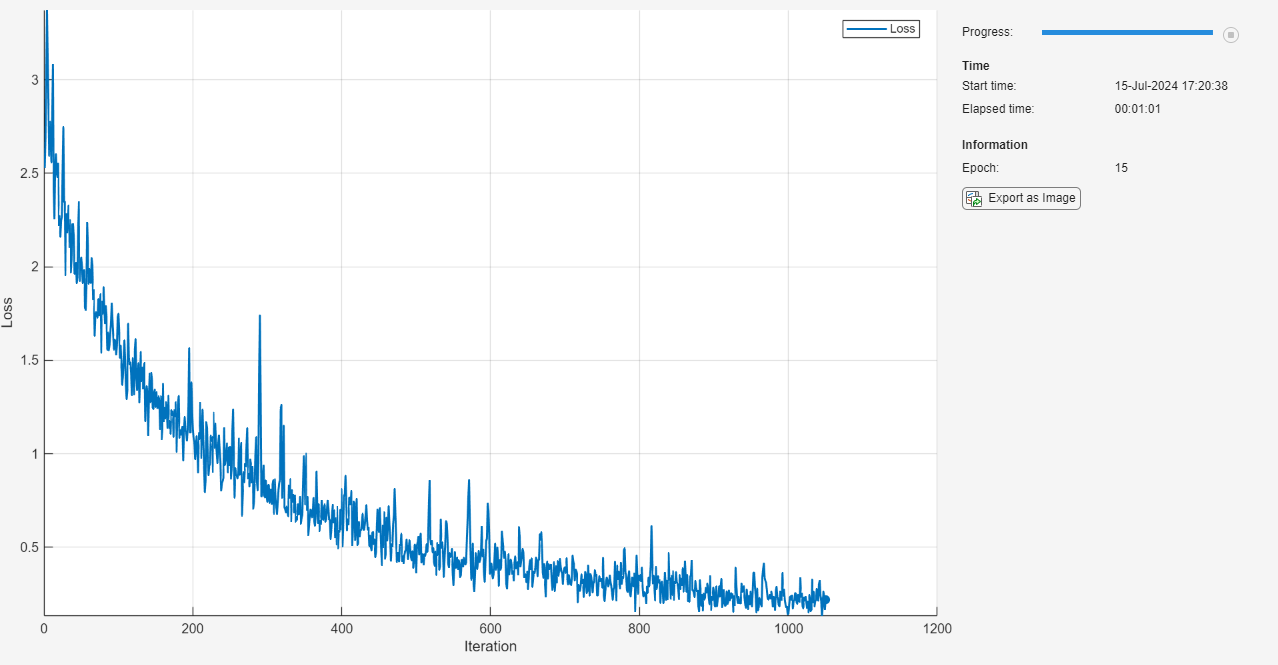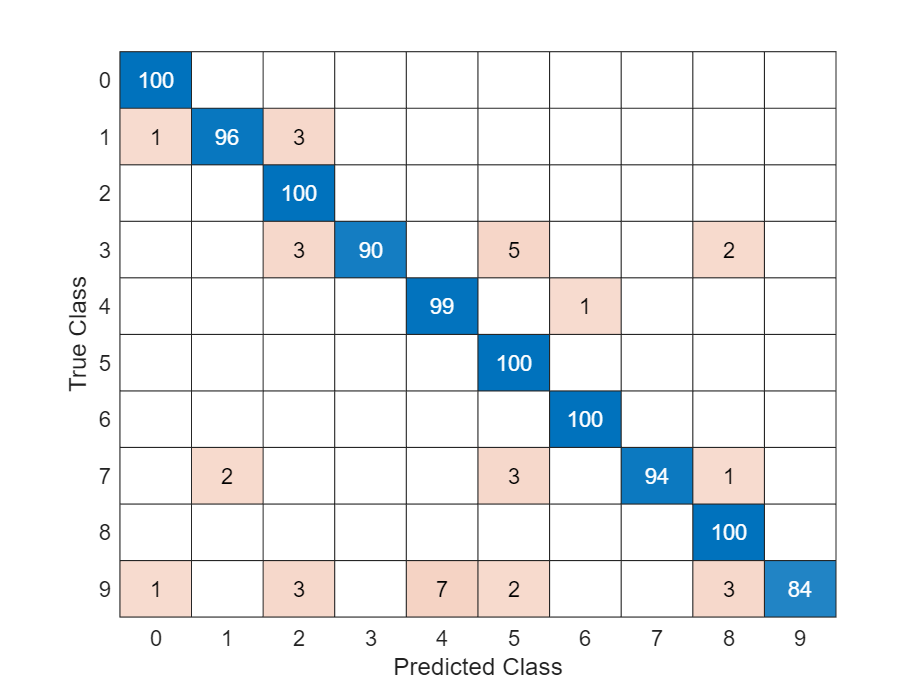# Train Network Using Custom Training Loop

This example shows how to train a network that classifies handwritten digits with a custom learning rate schedule.

You can train most types of neural networks using the `trainNetwork` and `trainingOptions` functions. If the `trainingOptions` function does not provide the options you need (for example, a custom learning rate schedule), then you can define your own custom training loop using `dlarray` and `dlnetwork` objects for automatic differentiation. For an example showing how to retrain a pretrained deep learning network using the `trainNetwork` function, see Transfer Learning Using Pretrained Network.

Training a deep neural network is an optimization task. By considering a neural network as a function $f\left(X;\theta \right)$, where $X$ is the network input, and $\theta$ is the set of learnable parameters, you can optimize $\theta$ so that it minimizes some loss value based on the training data. For example, optimize the learnable parameters $\theta$ such that for a given inputs $X$ with a corresponding targets $T$, they minimize the error between the predictions $Y=f\left(X;\theta \right)$ and $T$.

The loss function used depends on the type of task. For example:

• For classification tasks, you can minimize the cross entropy error between the predictions and targets.

• For regression tasks, you can minimize the mean squared error between the predictions and targets.

You can optimize the objective using gradient descent: minimize the loss $L$ by iteratively updating the learnable parameters $\theta$ by taking steps towards the minimum using the gradients of the loss with respect to the learnable parameters. Gradient descent algorithms typically update the learnable parameters by using a variant of an update step of the form ${\theta }_{t+1}={\theta }_{t}-\rho \nabla L$, where $t$ is the iteration number, $\rho$ is the learning rate, and $\nabla L$ denotes the gradients (the derivatives of the loss with respect to the learnable parameters).

This example trains a network to classify handwritten digits with the time-based decay learning rate schedule: for each iteration, the solver uses the learning rate given by ${\rho }_{\mathit{t}}=\frac{{\rho }_{0}}{1+\mathit{k}\text{\hspace{0.17em}}\mathit{t}}$, where t is the iteration number, ${\rho }_{0}$ is the initial learning rate, and k is the decay.

Load the digits data as an image datastore using the `imageDatastore` function and specify the folder containing the image data.

```unzip("DigitsData.zip") imds = imageDatastore("DigitsData", ... IncludeSubfolders=true, ... LabelSource="foldernames");```

Partition the data into training and validation sets. Set aside 10% of the data for validation using the `splitEachLabel` function.

`[imdsTrain,imdsValidation] = splitEachLabel(imds,0.9,"randomize");`

The network used in this example requires input images of size 28-by-28-by-1. To automatically resize the training images, use an augmented image datastore. Specify additional augmentation operations to perform on the training images: randomly translate the images up to 5 pixels in the horizontal and vertical axes. Data augmentation helps prevent the network from overfitting and memorizing the exact details of the training images.

```inputSize = [28 28 1]; pixelRange = [-5 5]; imageAugmenter = imageDataAugmenter( ... RandXTranslation=pixelRange, ... RandYTranslation=pixelRange); augimdsTrain = augmentedImageDatastore(inputSize(1:2),imdsTrain,DataAugmentation=imageAugmenter);```

To automatically resize the validation images without performing further data augmentation, use an augmented image datastore without specifying any additional preprocessing operations.

`augimdsValidation = augmentedImageDatastore(inputSize(1:2),imdsValidation);`

Determine the number of classes in the training data.

```classes = categories(imdsTrain.Labels); numClasses = numel(classes);```

### Define Network

Define the network for image classification.

• For image input, specify an image input layer with input size matching the training data.

• Do not normalize the image input, set the `Normalization` option of the input layer to `"none"`.

• Specify three convolution-batchnorm-ReLU blocks.

• Pad the input to the convolution layers such that the output has the same size by setting the `Padding` option to `"same"`.

• For the first convolution layer specify 20 filters of size 5. For the remaining convolution layers specify 20 filters of size 3.

• For classification, specify a fully connected layer with size matching the number of classes

• To map the output to probabilities, include a softmax layer.

When training a network using a custom training loop, do not include an output layer.

```layers = [ imageInputLayer(inputSize,Normalization="none") convolution2dLayer(5,20,Padding="same") batchNormalizationLayer reluLayer convolution2dLayer(3,20,Padding="same") batchNormalizationLayer reluLayer convolution2dLayer(3,20,Padding="same") batchNormalizationLayer reluLayer fullyConnectedLayer(numClasses) softmaxLayer];```

Create a `dlnetwork` object from the layer array.

`net = dlnetwork(layers)`
```net = dlnetwork with properties: Layers: [12×1 nnet.cnn.layer.Layer] Connections: [11×2 table] Learnables: [14×3 table] State: [6×3 table] InputNames: {'imageinput'} OutputNames: {'softmax'} Initialized: 1 View summary with summary. ```

### Define Model Loss Function

Training a deep neural network is an optimization task. By considering a neural network as a function $f\left(X;\theta \right)$, where $X$ is the network input, and $\theta$ is the set of learnable parameters, you can optimize $\theta$ so that it minimizes some loss value based on the training data. For example, optimize the learnable parameters $\theta$ such that for a given inputs $X$ with a corresponding targets $T$, they minimize the error between the predictions $Y=f\left(X;\theta \right)$ and $T$.

Create the function `modelLoss`, listed in the Model Loss Function section of the example, that takes as input the `dlnetwork` object, a mini-batch of input data with corresponding targets, and returns the loss, the gradients of the loss with respect to the learnable parameters, and the network state.

### Specify Training Options

Train for ten epochs with a mini-batch size of 128.

```numEpochs = 10; miniBatchSize = 128;```

Specify the options for SGDM optimization. Specify an initial learn rate of 0.01 with a decay of 0.01, and momentum 0.9.

```initialLearnRate = 0.01; decay = 0.01; momentum = 0.9;```

### Train Model

Create a `minibatchqueue` object that processes and manages mini-batches of images during training. For each mini-batch:

• Use the custom mini-batch preprocessing function `preprocessMiniBatch` (defined at the end of this example) to convert the labels to one-hot encoded variables.

• Format the image data with the dimension labels `"SSCB"` (spatial, spatial, channel, batch). By default, the `minibatchqueue` object converts the data to `dlarray` objects with underlying type `single`. Do not format the class labels.

• Train on a GPU if one is available. By default, the `minibatchqueue` object converts each output to a `gpuArray` if a GPU is available. Using a GPU requires Parallel Computing Toolbox™ and a supported GPU device. For information on supported devices, see GPU Computing Requirements (Parallel Computing Toolbox).

```mbq = minibatchqueue(augimdsTrain,... MiniBatchSize=miniBatchSize,... MiniBatchFcn=@preprocessMiniBatch,... MiniBatchFormat=["SSCB" ""], ... PartialMiniBatch="discard");```

Initialize the velocity parameter for the SGDM solver.

`velocity = [];`

Calculate the total number of iterations for the training progress monitor.

```numObservationsTrain = numel(imdsTrain.Files); numIterationsPerEpoch = floor(numObservationsTrain / miniBatchSize); numIterations = numEpochs * numIterationsPerEpoch;```

Initialize the `TrainingProgressMonitor` object. Because the timer starts when you create the monitor object, make sure that you create the object close to the training loop.

```monitor = trainingProgressMonitor( ... Metrics="Loss", ... Info=["Epoch" "LearnRate"], ... XLabel="Iteration");```

Train the network using a custom training loop. For each epoch, shuffle the data and loop over mini-batches of data. For each mini-batch:

• Evaluate the model loss, gradients, and state using the `dlfeval` and `modelLoss` functions and update the network state.

• Determine the learning rate for the time-based decay learning rate schedule.

• Update the network parameters using the `sgdmupdate` function.

• Update the loss, learn rate, and epoch values in the training progress monitor.

• Stop if the Stop property is true. The Stop property value of the `TrainingProgressMonitor` object changes to true when you click the Stop button.

```epoch = 0; iteration = 0; % Loop over epochs. while epoch < numEpochs && ~monitor.Stop epoch = epoch + 1; % Shuffle data. shuffle(mbq); % Loop over mini-batches. while hasdata(mbq) && ~monitor.Stop iteration = iteration + 1; % Read mini-batch of data. [X,T] = next(mbq); % Evaluate the model gradients, state, and loss using dlfeval and the % modelLoss function and update the network state. [loss,gradients,state] = dlfeval(@modelLoss,net,X,T); net.State = state; % Determine learning rate for time-based decay learning rate schedule. learnRate = initialLearnRate/(1 + decay*iteration); % Update the network parameters using the SGDM optimizer. [net,velocity] = sgdmupdate(net,gradients,velocity,learnRate,momentum); % Update the training progress monitor. recordMetrics(monitor,iteration,Loss=loss); updateInfo(monitor,Epoch=epoch,LearnRate=learnRate); monitor.Progress = 100 * iteration/numIterations; end end```### Test Model

Test the classification accuracy of the model by comparing the predictions on the validation set with the true labels.

After training, making predictions on new data does not require the labels. Create `minibatchqueue` object containing only the predictors of the test data:

• To ignore the labels for testing, set the number of outputs of the mini-batch queue to 1.

• Specify the same mini-batch size used for training.

• Preprocess the predictors using the `preprocessMiniBatchPredictors` function, listed at the end of the example.

• For the single output of the datastore, specify the mini-batch format `"SSCB"` (spatial, spatial, channel, batch).

```numOutputs = 1; mbqTest = minibatchqueue(augimdsValidation,numOutputs, ... MiniBatchSize=miniBatchSize, ... MiniBatchFcn=@preprocessMiniBatchPredictors, ... MiniBatchFormat="SSCB");```

Loop over the mini-batches and classify the images using `modelPredictions` function, listed at the end of the example.

`YTest = modelPredictions(net,mbqTest,classes);`

Evaluate the classification accuracy.

```TTest = imdsValidation.Labels; accuracy = mean(TTest == YTest)```
```accuracy = 0.9220 ```

Visualize the predictions in a confusion chart.

```figure confusionchart(TTest,YTest)```Large values on the diagonal indicate accurate predictions for the corresponding class. Large values on the off-diagonal indicate strong confusion between the corresponding classes.

### Supporting Functions

#### Model Loss Function

The `modelLoss` function takes a `dlnetwork` object `net`, a mini-batch of input data `X` with corresponding targets `T` and returns the loss, the gradients of the loss with respect to the learnable parameters in `net`, and the network state. To compute the gradients automatically, use the `dlgradient` function.

```function [loss,gradients,state] = modelLoss(net,X,T) % Forward data through network. [Y,state] = forward(net,X); % Calculate cross-entropy loss. loss = crossentropy(Y,T); % Calculate gradients of loss with respect to learnable parameters. gradients = dlgradient(loss,net.Learnables); end```

#### Model Predictions Function

The `modelPredictions` function takes a `dlnetwork` object `net`, a `minibatchqueue` of input data `mbq`, and the network classes, and computes the model predictions by iterating over all data in the `minibatchqueue` object. The function uses the `onehotdecode` function to find the predicted class with the highest score.

```function Y = modelPredictions(net,mbq,classes) Y = []; % Loop over mini-batches. while hasdata(mbq) X = next(mbq); % Make prediction. scores = predict(net,X); % Decode labels and append to output. labels = onehotdecode(scores,classes,1)'; Y = [Y; labels]; end end```

#### Mini Batch Preprocessing Function

The `preprocessMiniBatch` function preprocesses a mini-batch of predictors and labels using the following steps:

1. Preprocess the images using the `preprocessMiniBatchPredictors` function.

2. Extract the label data from the incoming cell array and concatenate into a categorical array along the second dimension.

3. One-hot encode the categorical labels into numeric arrays. Encoding into the first dimension produces an encoded array that matches the shape of the network output.

```function [X,T] = preprocessMiniBatch(dataX,dataT) % Preprocess predictors. X = preprocessMiniBatchPredictors(dataX); % Extract label data from cell and concatenate. T = cat(2,dataT{1:end}); % One-hot encode labels. T = onehotencode(T,1); end```

#### Mini-Batch Predictors Preprocessing Function

The `preprocessMiniBatchPredictors` function preprocesses a mini-batch of predictors by extracting the image data from the input cell array and concatenate into a numeric array. For grayscale input, concatenating over the fourth dimension adds a third dimension to each image, to use as a singleton channel dimension.

```function X = preprocessMiniBatchPredictors(dataX) % Concatenate. X = cat(4,dataX{1:end}); end```